# Power BI – SUM vs SUMX

SUM vs SUMX - Detail understanding of the difference between these two DAX functions

Introduction:

Let us have a quick and detailed understanding of the difference between SUM and SUMX DAX functions in Power BI.

Here I have considered the Bike Data where we have the Unit Price Details and Order Quantity details but the Sales value column is not part of the data set, so we will calculate the Sales using the Calculated Column as below.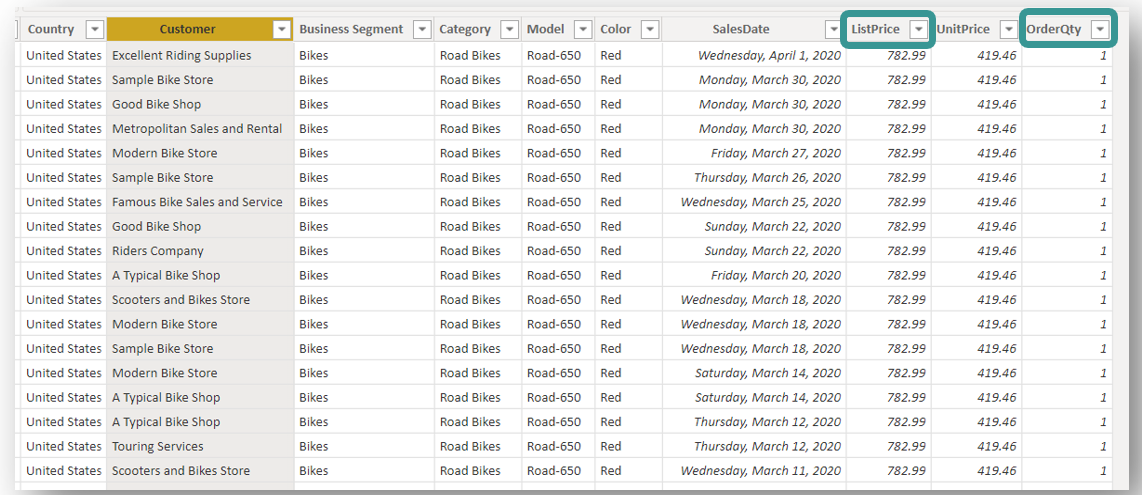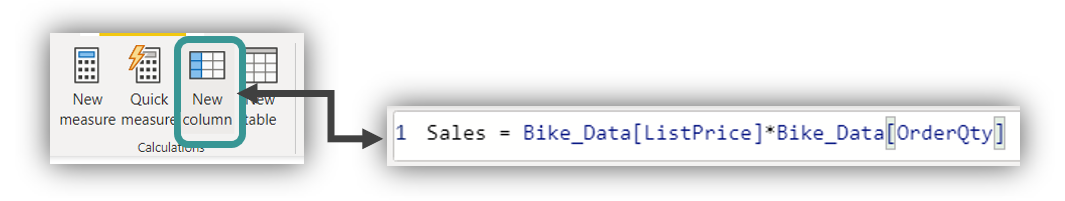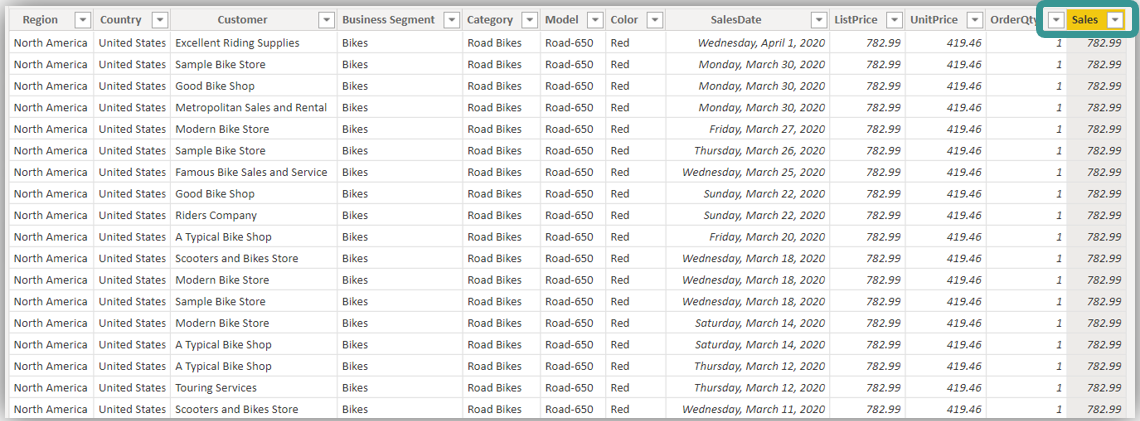SUM:

So, once we have a new column with Sales, it is easy to get the aggregate sales column by using below SUM function.

Syntax: SUM (COLUMN NAME)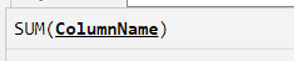where the column name must be an existing column from the data set. Let us take a Measure to calculate aggregate using the SUM function as below.

1 Total Sales = SUM (Bike_Data[Sales])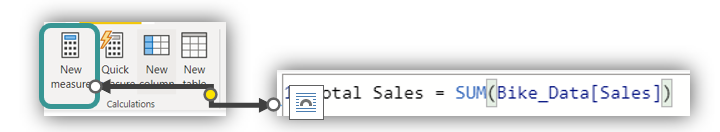Here in the above Total Sales is the Measure name, Bike_Data is the Data set name & Sales is the calculated column. This Measure will get the below Total Sales by using the SUM function, which will calculate the aggregate value of the whole column.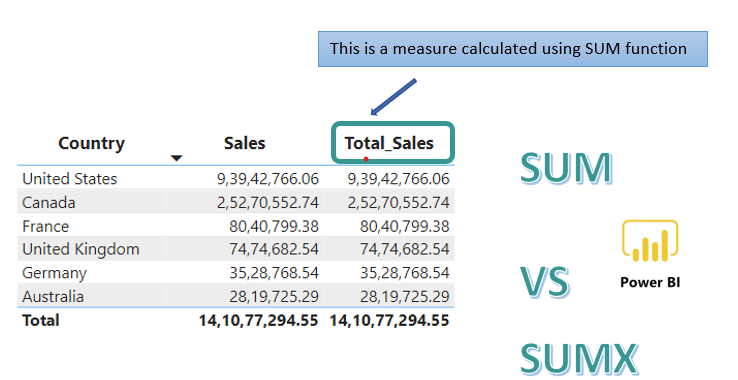SUMX:

If the calculated column insertion is not allowed i.e.., new column insertion to the data set is not possible, then the SUM function will not be a supportive function since this function will only work if we have an existing column from where the aggregate is calculated.

To solve this problem, Power BI has come up with a useful function called SUMX.

Before moving on, let us jump into a quick and short example.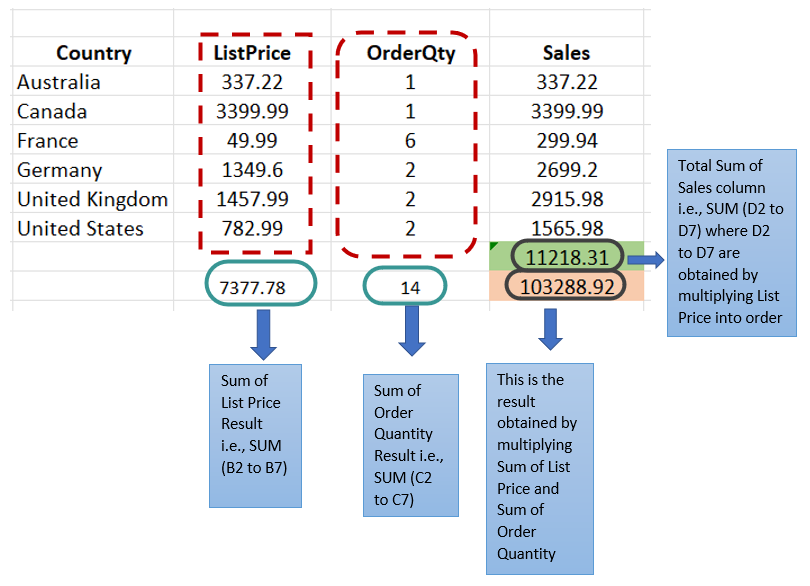In the above example, if we take the sum of Order Quantity and list Price and then multiply these two results, will that provide us the correct Aggregate of Sales? The answer will be “NO” since taking the sum column by column and multiplying will not give the right results. Instead, the row-by-row value needs to be multiplied initially and the values which we get need to be aggregated.

Let us implement the above example using the SUM function in our Bike_Data data set. Below is the new Measure where the SUM function is initially implemented on List Price and then on Order Quantity. These aggregated values are finally multiplied, where values are obtained as shown below under the Total Sales 2 column. This is incorrect as compared to the Total Sales column, where the Total Sales column is earlier calculated using the SUM function through an existing sales column.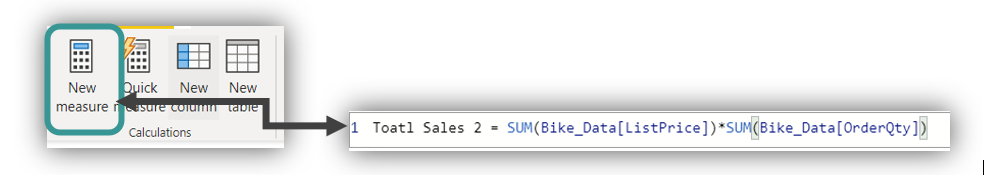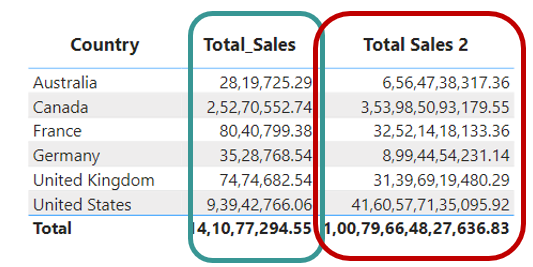So, from the above example, it can be understood that row-by-row calculation is required if we do not have a predefined or existing column in a Data set in Power BI. This is where SUMX would be a handy function for us.

Let us see the syntax of SUMX

Syntax: SUMX (Table, Expression).Here the Table refers to the Table name where expression needs to be applied. On the other hand, Expression is a mathematical operation to be performed row by row and then aggregate the whole value.

So, in the Bike_Data data set example, the SUMX syntax can be applied as below by taking New Measure.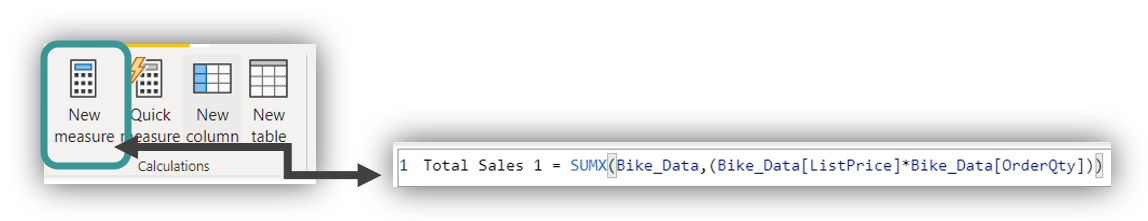Here, the SUMX function will first evaluate the expression and then aggregate it.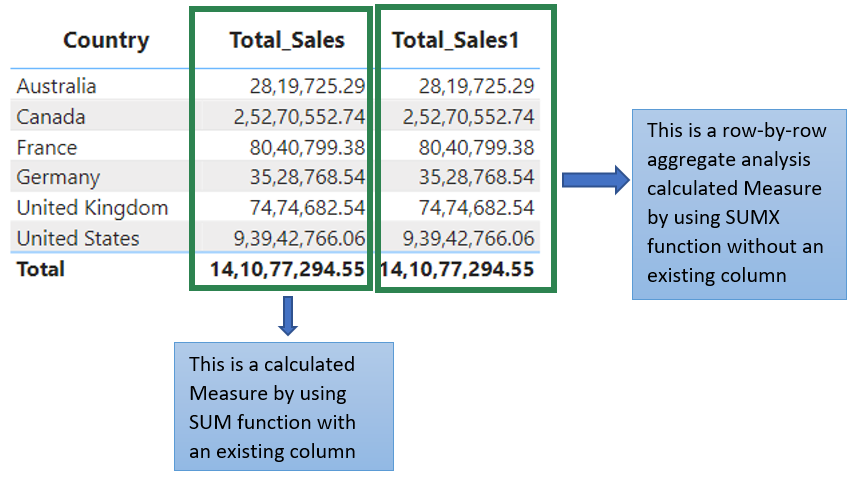SUMMARY:

To summarize, a SUM is an aggregate Measure. We can calculate the total SUM for an existing column in the data set. In contrast, SUMX function will calculate the sum without even having an existing column by giving an expression, where this expression will evaluate the values row by row. Then it aggregates it, which gives the same result as SUM.Miss. Priya
Business analyst, Blogger and Tableau Trainer.